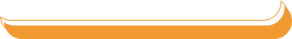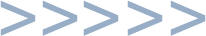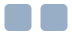PHYSICS  HONORS OUTLINE
Measurement - Units, Uncertainty, Graphical Analysis
Science Skills Assessment - Analyze your skills and then consult this guide
In-Class Practice
Graphing - directions, examples, free graph paper
Notes - uncertainty, significant digits, and units
Functions Overview - summary of important types of equations
Review Problems

Kinematics - a Mathematical Description of Motion
Objectives & Homework - Kinematics
In-Class Practice - Word Problems
In-Class Practice - Position Graphs
In-Class Practice - Velocity Graphs
Review Problems
Lab Report - Freefall Acceleration

Vectors - Two-Dimensional Kinematics
Objectives & Homework - Vectors
In-Class Practice
Review Problems
Lab Report - Projectile Motion

Forces - Newton's 3 Laws of Motion (Dynamics)
Objectives & Homework - Forces
In-Class Practice
net Force - understanding and practice
Review Problems
Lab Report - Newton's 2nd Law

Periodic Motion & Gravity - SHM, Orbits, & Force Fields
Objectives & Homework - Periodic Motion
Solar System Data - Sun, planets, moons
In-Class Practice
SHM Notes basic info on Simple Harmonic Motion
Review Problems
Lab Report - the Conical Pendulum

Conservation of Energy & Momentum - an Alternate Form of Mechanics
Objectives & Homework - Energy
Objectives & Homework -
In-Class Practice - Energy
In-Class Practice - Momentum
Energy Form Examples
Review Problems
Lab Report - Mass on a Spring

Electricity - Electrostatics, Coulomb's Law, & Simple Circuits
Objectives & Homework - Electrostatics
Objectives & Homework - Current & Circuits
In-Class Practice
The Digital Multimeter - a Primer
Review Problems
Some Notes
Lab Report - Virtual Circuits

Waves & Interference - Sound, Light, etc.
Objectives & Homework - Waves
Objectives & Homework - Interference
Wave Notes - Basic Definitions and Formulas
Notes on Sound - Defining Properties
Notes on EMR - Defining Properties of Light
Notes on Wave Graphs - Two Types and Parameters
Standing Waves Notes - Definitions and Formulas
Electromagnetic Radiation - Information and Practice
In-Class Practice
Review Problems
Lab Report - Virtual Waves on a String

Final Exam!
About the Final Exam . . .
Review Questions and Problems - Multiple Choice